# Numerically solving Time-independent Schrödinger eqn. using Shooting algorithm

Gold Member
2020 Award
I have to solve the 1D Time-independent Schrödinger equation (TISE) using the shooting algorithm. As far as I understood from this video on Shooting method for solving BVP, I will have to solve the problem by using IVP solvers (like RK2 or RK4 methods), and guess a value for the derivative of the function at the starting point. Then, I iterate over to the other end point. I already know the value of the function at the other end point, and I compare the numerically obtained value with the known value, and modify my guess accordingly.

I can write the 1D TISE as $$\psi''(x) = -k^2(x) \psi(x),$$ where $$k^2(x) = \dfrac{2m}{\hbar^2} \left[ E - V(x) \right].$$ Since the TISE is an eigenvalue equation, I do not know the value of E beforehand, and I have to guess it. So, I have to make two guesses — ##\psi'(x = 0)## and ##E##. Now, suppose I have been given ##\psi(x = 0)## and ##\psi(x = x_N)##, and, of course, I know the form of ##V(x)##. After one iteration from ##x = 0 \text{ to } x_N##, I find that the numerically computed ##\psi(x_N)## doesn't quite match the given value. Now, which one do I change — ##\psi'(x = 0)## or ##E##?

Chestermiller
Mentor
I have to solve the 1D Time-independent Schrödinger equation (TISE) using the shooting algorithm. As far as I understood from this video on Shooting method for solving BVP, I will have to solve the problem by using IVP solvers (like RK2 or RK4 methods), and guess a value for the derivative of the function at the starting point. Then, I iterate over to the other end point. I already know the value of the function at the other end point, and I compare the numerically obtained value with the known value, and modify my guess accordingly.

I can write the 1D TISE as $$\psi''(x) = -k^2(x) \psi(x),$$ where $$k^2(x) = \dfrac{2m}{\hbar^2} \left[ E - V(x) \right].$$ Since the TISE is an eigenvalue equation, I do not know the value of E beforehand, and I have to guess it. So, I have to make two guesses — ##\psi'(x = 0)## and ##E##. Now, suppose I have been given ##\psi(x = 0)## and ##\psi(x = x_N)##, and, of course, I know the form of ##V(x)##. After one iteration from ##x = 0 \text{ to } x_N##, I find that the numerically computed ##\psi(x_N)## doesn't quite match the given value. Now, which one do I change — ##\psi'(x = 0)## or ##E##?
You vary E. The arbitrary value of ##\psi '(0)## will just change the amplitude of the solution. So just use a value of 1.0 for it in all cases.

Last edited:
•Wrichik Basu
Gold Member
2020 Award
You vary E. The arbitrary value of ##\psi '(0)## will just change the amplitude of the solution. So just use a value of 1.0 for it in all cases.
Thanks.

Based on this, I wrote a MATLAB program. I don't know if I should be heading to the MATLAB forum for posting the code, but since it's computational physics, let me post here itself:
Matlab:
function shootingAlgo(psi0, x0, xEnd, m, Vx, E)

hbar = 1;
k = @(x) (2.*m./(hbar.^2)) .* (E - Vx(x));

[xVal, psiVal] = ode45(@schrod, [x0 xEnd], [1.0; psi0]);

hold off;
plot(xVal, psiVal(:, 2), '.r');
hold on; grid on; grid minor;
plot(xVal, psiVal(:, 2), '-b');

function dPsi_dt = schrod(x, psi)
dPsi_dt = [psi(2); -(k(x)).^2 .* psi(1)];
end

end
If I took the actual value of ##\hbar##, then the computation never ended. I read somewhere on the net (can't find it now) that taking ##\hbar = 1## will work. The function won't automatically vary ##E##; you would have to do it yourself from the command line. I called the function from command line as follows:
Matlab:
>> shootingAlgo(0, 0, 2, 2, @Vx, 0.392)
where Vx is the infinite square well potential:
Matlab:
function V = Vx(x)

L = 2;
if (0 <= x) && (x <= L)
V = 0;
else
V = 9999999;
end

end
For ##E = 0.392##, I get this figure: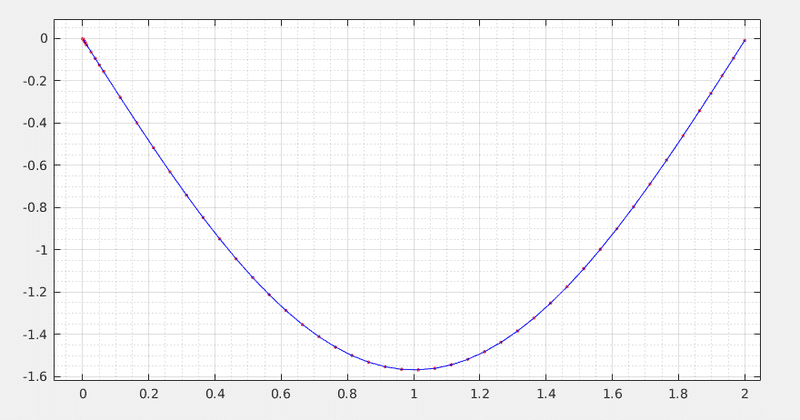Apparently, this is the same figure for the first stationary state, but with the graph inverted. Any idea what I am doing wrong?

Edit: Code and figure generated by it didn't match; edited to fix it.

Last edited:
Paul Colby
Gold Member
Any idea what I am doing wrong?
Looks good. The phase or sign of ##\psi## is arbitrary. You are free to choose it.

Oh, you plotted ##-psiVal## BTW

Gold Member
2020 Award
Looks good. The phase or sign of ψ is arbitrary. You are free to choose it.
Okay, thanks.
Oh, you plotted ##-psiVal## BTW
My bad; I was debugging, and plotting -psiVal gave me the correct graph. I have edited the code.

Paul Colby
Gold Member
The slope ##\psi’(0) \lt 0## yet you’ve set it to 1. Why is this?

Gold Member
2020 Award
The slope ##\psi’(0) \lt 0## yet you’ve set it to 1. Why is this?
No idea. Even I was wondering why this happened.

Paul Colby
Gold Member
I would look at how the initial conditions for your integrator are set up. ode45 is told what the initial slope is. You must have set this up incorrectly. It should be 1 but it comes back as -1. My bet is your problem is there. It's worth figuring out.

•Wrichik Basu
Gold Member
2020 Award
I would look at how the initial conditions for your integrator are set up. ode45 is told what the initial slope is. You must have set this up incorrectly. It should be 1 but it comes back as -1. My bet is your problem is there. It's worth figuring out.
I sent the program to our college Professor, and he explained that the eigenfunctions are defined only upto a constant, and the Schrödinger equation cannot distinguish between ##\psi## and ##\mathrm{constant} \times \psi##. In standard textbooks, this constant is positive, but in my case, due to the initial values, it is negative. But the program is fine.

Paul Colby
Gold Member
But the program is fine.
Yeah, I’m still scratching my head on this one. I copied your code and used octave. Got exactly your result. I need to refactor it a bit so I can check each piece. My current theory is you are plotting ##-k(x)^2\psi## which will have a negative sign but this needs proof first.

Gold Member
2020 Award
If anybody is still interested, the complete function is this:
Matlab:
function [E, xVal, normalPsi] = TISE_shootingAlgo(psi0, psiEnd, x0, xEnd, m, Vx, E_guess, error)
%
% TISE_shootingAlgo Solves the time-independent Schrodinger equation using the shooting algorithm.
%
% To find a numerical solution of the differential equation, MATLAB's ode45 is used.
%
% The first energy eigenvalue greater than or equal to the given E_guess will be computed.
%
%   USAGE:
%     TISE_shootingAlgo(psi0, psiEnd, x0, xEnd, m, Vx, E_guess, error)
%
%   INPUTS:
%     → psi0 -  - The boundary value of ψ at x0.
%     → psiEnd  - The boundary value of ψ at xEnd.
%     → x0 - -  - The starting value of position coordinate.
%     → xEnd -  - The last value position coordinate.
%     → m -  -  - The mass.
%     → Vx - -  - The potential as a function of x. Should be a callable function.
%     → E_guess - The initial guess for the energy eigenvalue. The first eigenvalue greater than
%                 or equal to this value will be computed.
%     → error - - The maximum error permissible for calculation of energy eigenvalue.
%
%   RETURNS:
%     → The energy eigenvalue >= E_guess.
%     → The values of x.
%     → The corresponding values of normalized wave function.
%

hbar = 1;
k = @(x, E) (2.*m./(hbar.^2)) .* (E - Vx(x));

E = E_guess;  % We start with the given guess of energy
dE = 0.2;

% First computation:
[xVal, psiVal] = ode45(@odefun, [x0 xEnd], [1.0; psi0]);

psiEnd_old = psiVal(end);

while abs(dE) > error

E = E + dE;

[xVal, psiVal] = ode45(@odefun, [x0 xEnd], [1.0; psi0]);

psiEnd_new = psiVal(end);

% Check if current psiVal(end) and old psiVal(end) are on opposite sides of psiEnd:
if (psiEnd - psiEnd_old) * (psiEnd - psiEnd_new) < 0

% If yes, change the sign and decrease the magnitude of dE:
dE = -dE / 2;

end

psiEnd_old = psiEnd_new;

end

% Normalize ψ:
normalPsi = normalizePsi(xVal, psiVal(:, 2));

function dPsi_dt = odefun(x, psi)
dPsi_dt = [psi(2); -(k(x, E)).^2 .* psi(1)];
end

function normalPsi = normalizePsi(xVal, psiVal)

I = trapz(xVal, abs(psiVal).^2);
A = sqrt(1/I);
normalPsi = A .* psiVal;

end

end
You can, for example, execute a simple script calling the function to solve the TISE:
Matlab:
%% Harmonic Oscillator

psi0 = 0;
psiEnd = 0;
x0 = -2;
xEnd = 2;
m = 0.5;
E_guess = 2.8;
maxError = 1e-4;

hold off;
[E, xVal, normalPsi] = TISE_shootingAlgo(psi0, psiEnd, x0, xEnd, m, @Vx_oscillator, E_guess, maxError);
plot(xVal, normalPsi, '-o', 'DisplayName', ['E = ' num2str(E)]);
grid on; grid minor;
xlim([x0-0.1 xEnd+0.1]);
ylim([-1.1 1.1]);
legend('Interpreter', 'latex')
hold on;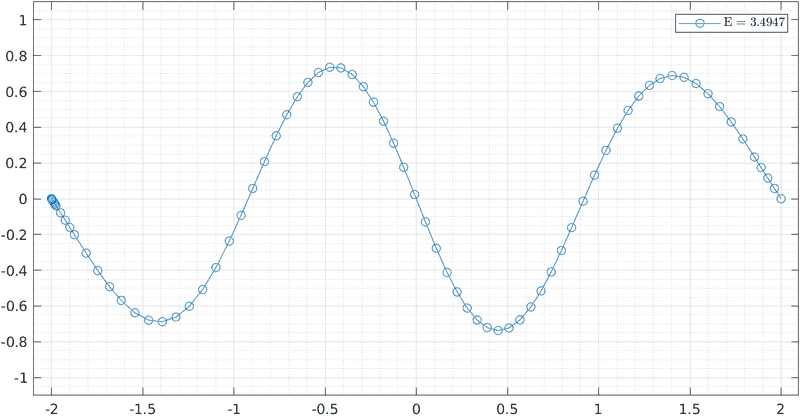The inverting problem is still there, by the way.

Paul Colby
Gold Member
I’m still confused by the initial condition given in line 36 of function TISE_shootingAlgo. One uses a vector containing the current value of ##\psi## as the first element and ##\psi’## as the second. Your initial value given on line 36 is,

##\left(\begin{array}{c} \psi \\ \psi’\end{array}\right) =\left(\begin{array}{c} 1\\ 0\end{array}\right) ##

which appears like the components are switched. Am I reading this wrong?

••BvU and Wrichik Basu
Gold Member
2020 Award
I’m still confused by the initial condition given in line 36 of function TISE_shootingAlgo. One uses a vector containing the current value of ##\psi## as the first element and ##\psi’## as the second. Your initial value given on line 36 is,

##\left(\begin{array}{c} \psi \\ \psi’\end{array}\right) =\left(\begin{array}{c} 1\\ 0\end{array}\right) ##

which appears like the components are switched. Am I reading this wrong?
No, I was wrong. You found the mistake. Even lines 46 and 38 in the function file are wrong. Both have to be corrected to get the result. Thanks a ton for pointing out the mistake that even my Prof. couldn't find; I don't have words to thank you.

The corrected code is:
Matlab:
function [E, xVal, normalPsi] = TISE_shootingAlgo(psi0, psiEnd, x0, xEnd, m, Vx, E_guess, error)
%
% TISE_shootingAlgo Solves the time-independent Schrodinger equation using the shooting algorithm.
%
% To find a numerical solution of the differential equation, MATLAB's ode45 is used.
%
% The first energy eigenvalue greater than or equal to the given E_guess will be computed.
%
%   USAGE:
%     TISE_shootingAlgo(psi0, psiEnd, x0, xEnd, m, Vx, E_guess, error)
%
%   INPUTS:
%     → psi0 -  - The boundary value of ψ at x0.
%     → psiEnd  - The boundary value of ψ at xEnd.
%     → x0 - -  - The starting value of position coordinate.
%     → xEnd -  - The last value position coordinate.
%     → m -  -  - The mass.
%     → Vx - -  - The potential as a function of x. Should be a callable function.
%     → E_guess - The initial guess for the energy eigenvalue. The first eigenvalue greater than
%                 or equal to this value will be computed.
%     → error - - The maximum error permissible for calculation of energy eigenvalue.
%
%   RETURNS:
%     → The energy eigenvalue >= E_guess.
%     → The values of x.
%     → The corresponding values of normalized wave function.
%

hbar = 1;
k = @(x, E) (2.*m./(hbar.^2)) .* (E - Vx(x));

E = E_guess;  % We start with the given guess of energy
dE = 0.2;

% First computation:
[xVal, psiVal] = ode45(@odefun, [x0 xEnd], [psi0; 1.0]);

psiEnd_old = psiVal(end, 1);

while abs(dE) > error

E = E + dE;

[xVal, psiVal] = ode45(@odefun, [x0 xEnd], [psi0; 1.0]);

psiEnd_new = psiVal(end, 1);

% Check if current psiVal(end) and old psiVal(end) are on opposite sides of psiEnd:
if (psiEnd - psiEnd_old) * (psiEnd - psiEnd_new) < 0

% If yes, change the sign and decrease the magnitude of dE:
dE = -dE / 2;

end

psiEnd_old = psiEnd_new;

end

% Normalize ψ:
normalPsi = normalizePsi(xVal, psiVal(:, 1));

function dPsi_dt = odefun(x, psi)
dPsi_dt = [psi(2); -(k(x, E)).^2 .* psi(1)];
end

function normalPsi = normalizePsi(xVal, psiVal)

I = trapz(xVal, abs(psiVal).^2);
A = sqrt(1/I);
normalPsi = A .* psiVal;

end

end
Using this function and the script file posted earlier, I get the following correct graph: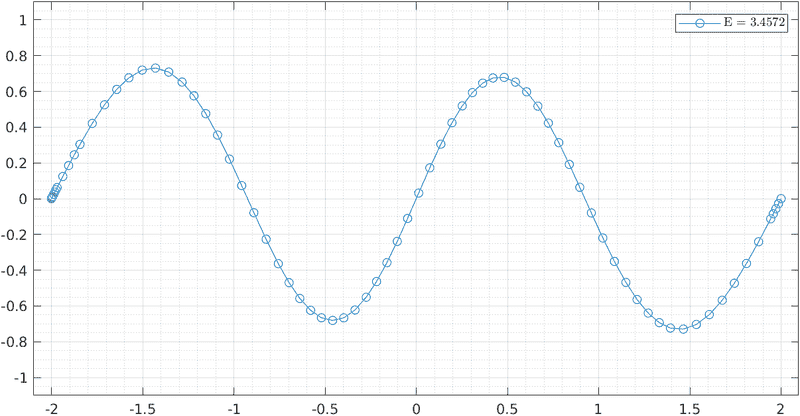bob012345
Gold Member
You are showing what looks like the ground state waveform and the third excited state or fourth energy level. What did you compute for the ground state energy? Are the two energy levels in the proper ratio of 16 to 1?

Here is a reference to using the Shooting Method on your problem with numerical results.

https://www1.itp.tu-berlin.de/brandes/public_html/qm/qv3.pdf

This reference has a finite well problem but I think the way they find the roots is more clear.

https://physics.weber.edu/schroeder/quantum/Numerical.pdf

Last edited:
Gold Member
2020 Award
You are showing what looks like the ground state waveform and the third excited state or fourth energy level. What did you compute for the ground state energy? Are the two energy levels in the proper ratio of 16 to 1?
There can be several problems here. My function can be simply wrong. But I am using ode45, which won't return a wrong result. So, the way I am varying the energy such that psi(xEnd) is as close to 0 as possible might be wrong. Or, the potential function (which I forgot to post earlier) itself might be wrong because I devised it myself.
Matlab:
psi0 = 0;
psiEnd = 0;
x0 = -2;
xEnd = 2;
m = 0.5;
E_guess = 3.3;
maxError = 1e-4;

hold off;
[E, xVal, normalPsi] = TISE_shootingAlgo(psi0, psiEnd, x0, xEnd, m, @Vx_oscillator, E_guess, maxError);
plot(xVal, normalPsi, '-o', 'DisplayName', ['E = ' num2str(E)]);
grid on; grid minor;
xlim([x0-0.1 xEnd+0.1]);
ylim([-1.1 1.1]);
legend('Interpreter', 'latex')
hold on;

function V = Vx_oscillator(x)

m = 0.5;
omega = 1;
V = 0.5 .* m .* omega.^2 * x.^2;

end

bob012345
Gold Member
I thought this was an infinite square well. Your potential looked correct. My question is about the assumption of X0. You have X0=-2 but maybe the program assumes X0=0?

Since Energy =##n^2 \pi^2/L^2## for a total well width of 4 as you have the energies are ## \pi^2/16## for the ground state and ## \pi^2## for the fourth state you show. If you can get the correct ground state the others will work out.

Last edited:
Gold Member
2020 Award
For the infinite square well potential, I am using the following:
Matlab:
psi0 = 0;
psiEnd = 0;
x0 = 0;
xEnd = 2;
m = 2;
E_guess = 0;
maxError = 1e-10;

hold off;

[E, xVal, normalPsi] = TISE_shootingAlgo(psi0, psiEnd, x0, xEnd, m, @Vx_infSqWell, E_guess, maxError);

plot(xVal, normalPsi, '-o', 'DisplayName', ['E = ' num2str(E)]);

grid on; grid minor;
hold on;

xlim([x0-0.1 xEnd+0.1]);
ylim([-1.1 1.1]);
legend('Interpreter', 'latex');

function V = Vx_infSqWell(x)

L = 2;
if (0 <= x) && (x <= L)
V = 0;
else
V = 9999999;
end

end
and I get this graph: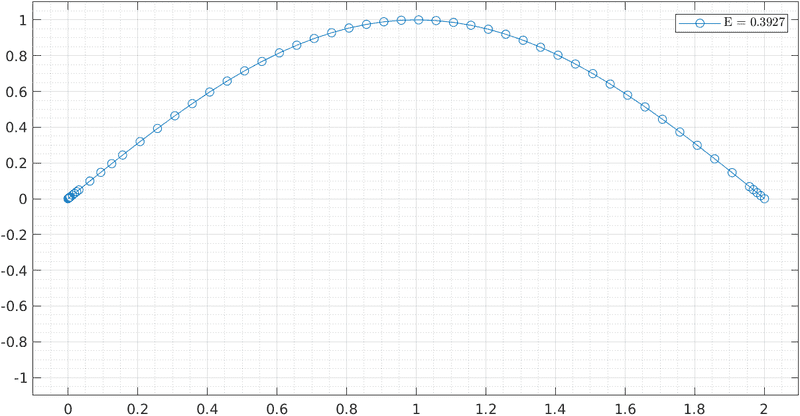Analytically, as you said, it should be 0.61685. The value calculated from my program is way off. I am not sure where the problem is occurring.

bob012345
Gold Member
For the infinite square well potential, I am using the following:
Matlab:
psi0 = 0;
psiEnd = 0;
x0 = 0;
xEnd = 2;
m = 2;
E_guess = 0;
maxError = 1e-10;

hold off;

[E, xVal, normalPsi] = TISE_shootingAlgo(psi0, psiEnd, x0, xEnd, m, @Vx_infSqWell, E_guess, maxError);

plot(xVal, normalPsi, '-o', 'DisplayName', ['E = ' num2str(E)]);

grid on; grid minor;
hold on;

xlim([x0-0.1 xEnd+0.1]);
ylim([-1.1 1.1]);
legend('Interpreter', 'latex');

function V = Vx_infSqWell(x)

L = 2;
if (0 <= x) && (x <= L)
V = 0;
else
V = 9999999;
end

end
and I get this graph:

View attachment 285366

Analytically, as you said, it should be 0.61685. The value calculated from my program is way off. I am not sure where the problem is occurring.
Can you do a couple of hand iterations of you algorithm?
For the infinite square well potential, I am using the following:
Matlab:
psi0 = 0;
psiEnd = 0;
x0 = 0;
xEnd = 2;
m = 2;
E_guess = 0;
maxError = 1e-10;

hold off;

[E, xVal, normalPsi] = TISE_shootingAlgo(psi0, psiEnd, x0, xEnd, m, @Vx_infSqWell, E_guess, maxError);

plot(xVal, normalPsi, '-o', 'DisplayName', ['E = ' num2str(E)]);

grid on; grid minor;
hold on;

xlim([x0-0.1 xEnd+0.1]);
ylim([-1.1 1.1]);
legend('Interpreter', 'latex');

function V = Vx_infSqWell(x)

L = 2;
if (0 <= x) && (x <= L)
V = 0;
else
V = 9999999;
end

end
and I get this graph:

View attachment 285366

Analytically, as you said, it should be 0.61685. The value calculated from my program is way off. I am not sure where the problem is occurring.
The first plot of the ground state goes from 0 to 2 but the second plot of the ##n=4## plot goes from -2 to 2.

I suggest seeing if you can go through an iteration of the algorithm by hand if you have the actual code to follow.

Gold Member
2020 Award
Can you do a couple of hand iterations of you algorithm?

The first plot of the ground state goes from 0 to 2 but the second plot of the ##n=4## plot goes from -2 to 2.

I suggest seeing if you can go through an iteration of the algorithm by hand if you have the actual code to follow.
I think you are confusing post #13 with #17. The former is for the harmonic oscillator, while the latter is for the infinite square well.

For the infinite square well, here is a graph of the eigenfunctions and the corresponding energy eigenvalues for different levels: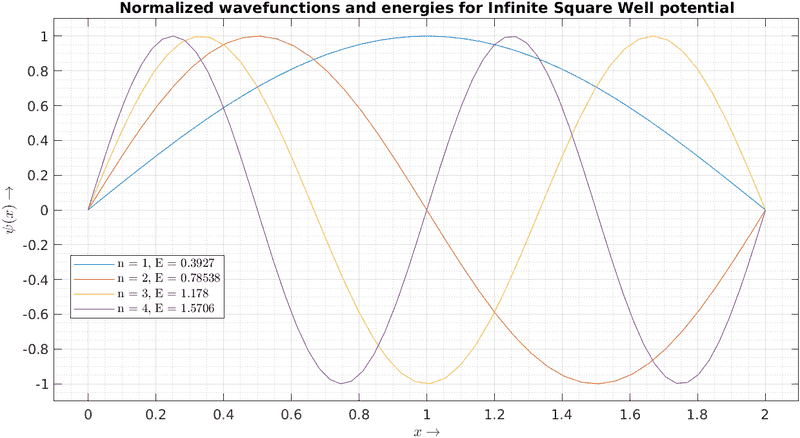I agree that the energy eigenvalues don't match the analytical values. And I don't have a clue where the error is.

bob012345
Gold Member
I'm going to answer the for the case of the infinite square well with a total well width of 2 as shown above by you. Thus the energies should be ##n^2 \pi^2/8ma^2## where the well goes from -a to +a so in this case a=1. Therefore the energies are ##n^2 \pi^2/4## since m=0.5 as specified above. The ground state energy should be 2.4674 now.

Looking at your numbers for the infinite square well, the ground state is off by almost exactly a factor of ##2\pi##. Then, the energies should scale as ##n^2## and not ##n## as your energies scale above. These are clues as to where to look.

Last edited:
•Wrichik Basu
Gold Member
2020 Award
Eureka! Found the problem! In the code I posted in post #13, line 30 is wrong. It should be:
k = @(x, E) sqrt(2 * m * (E - Vx(x))) ./ hbar;
For the infinite square well, with L = 1 and m = 1, now I get: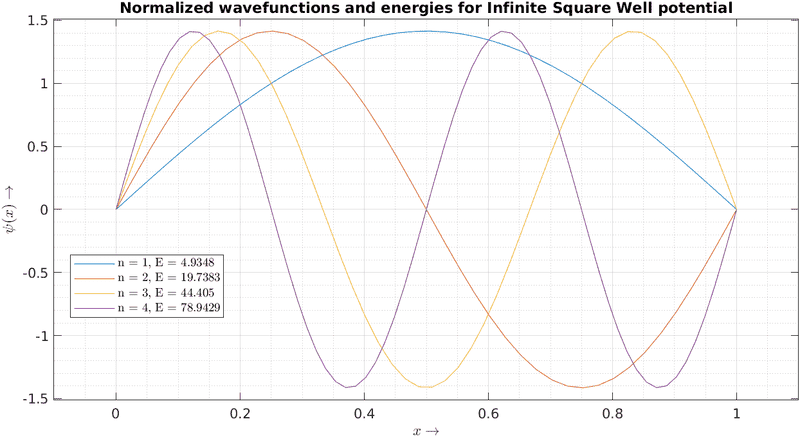•bob012345, BvU and Paul Colby